Home
Hostname: page-component-888d5979f-6thx7 Total loading time: 0.159 Render date: 2021-10-25T15:16:13.862Z Has data issue: true Feature Flags: { "shouldUseShareProductTool": true, "shouldUseHypothesis": true, "isUnsiloEnabled": true, "metricsAbstractViews": false, "figures": true, "newCiteModal": false, "newCitedByModal": true, "newEcommerce": true, "newUsageEvents": true }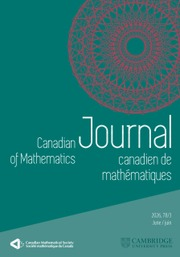Canadian Journal of Mathematics

# Galois Representations with Non-Surjective Traces

Published online by Cambridge University Press:  20 November 2018

## Abstract

HTML view is not available for this content. However, as you have access to this content, a full PDF is available via the ‘Save PDF’ action button.

Let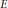$E$ be an elliptic curve over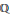$\mathbb{Q}$ , and let$r$ be an integer. According to the Lang-Trotter conjecture, the number of primes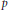$p$ such that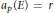${{a}_{p}}\left( E \right)=r$ is either finite, or is asymptotic to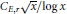${{C}_{E,r}}\sqrt{x}/\log x$ where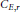${{C}_{E,r}}$ is a non-zero constant. A typical example of the former is the case of rational$\ell$ -torsion, where${{a}_{p}}\left( E \right)=r$ is impossible if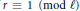$r\equiv 1\,\left( \bmod \,\ell \right)$ . We prove in this paper that, when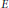$E$ has a rational$\ell$ -isogeny and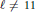$\ell \ne 11$ , the number of primes$p$ such that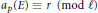${{a}_{p}}\left( E \right)\equiv r\,\left( \bmod \,\ell \right)$ is finite (for some$r$ modulo$\ell$ ) if and only if$E$ has rational$\ell$ -torsion over the cyclotomic field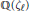$\mathbb{Q}\left( {{\zeta }_{\ell }} \right)$ . The case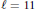$\ell =11$ is special, and is also treated in the paper. We also classify all those occurences.

## Keywords

Type
Research Article
Information
Canadian Journal of Mathematics , 01 October 1999 , pp. 936 - 951

## References

 Modular functions of one variable VI (eds. Serre, J.-P. and Zagier, D. B.). Proceedings of the Second International Conference, held at the University of Bonn, Bonn, July 2–14, 1976. Lecture Notes in Math. 627, Springer-Verlag, Berlin-New York, 1977.Google Scholar
 Batut, C., Belabas, K., Bernardi, D., Cohen, H. and Olivier, M., PARI-GP. Google Scholar
 Coleman, R., Effective Chabauty. Duke Math. J. 52(1985), 765770.CrossRefGoogle Scholar
 Cremona, J. E., Algorithms for modular elliptic curves. Cambridge University Press, 1992.Google Scholar
 David, C. and Pappalardi, F., Average Frobenius distributions of elliptic curves. Internat. Math. Res. Notices 4(1999), 165183.CrossRefGoogle Scholar
 Deuring, M., Die Typen derMultiplikatorenringe elliptischer Funktionenkörper. Abh.Math. Sem. Hansischen Univ. 14(1941), 197272.CrossRefGoogle Scholar
 Duke, W., Rational elliptic curves with no exceptional primes. C. R. Acad. Sci. Paris 325(1997), 813818.CrossRefGoogle Scholar
 Grant, D., A formula for the number of elliptic curves with exceptional primes. Compositio Math., to appear.Google Scholar
 Lang, S. and Trotter, H., Frobenius distributions in GL2-extensions. Lecture Notes in Math. 504, Springer-Verlag, 1976.Google Scholar
 Mazur, B., Modular curves and the Eiseinstein ideal. Inst. Hautes Études Sci. Publ. Math. 47(1977), 33186.CrossRefGoogle Scholar
 Mazur, B., Rational isogenies of prime degree. Invent.Math. 44(1978), 129162.CrossRefGoogle Scholar
 Mazur, B. and Swinnerton-Dyer, P., Arithmetic of Weil curves. Invent.Math. 25(1974), 161.CrossRefGoogle Scholar
 Momose, F., p-torsion points on elliptic curves defined over quadratic fields. Nagoya Math. J. 96(1984), 115137.CrossRefGoogle Scholar
 Ogg, A. P., Rational points on certain elliptic modular curves. Proc. Symp. Pure Math. 24(1973), 221231.CrossRefGoogle Scholar
 Serre, J.-P., Propriétés galoisiennes des points d’ordre fini des courbes elliptiques. Invent.Math. 15(1972), 259331.CrossRefGoogle Scholar
 Silverman, J., The arithmetic of elliptic curves. Springer-Verlag, 1986.CrossRefGoogle Scholar
 Stark, H., Counting points on CM elliptic curves. Rocky Mountain J. Math. 26(1996), 11151138.CrossRefGoogle ScholarYou have Access
2
Cited by

# Send article to Kindle

Note you can select to send to either the @free.kindle.com or @kindle.com variations. ‘@free.kindle.com’ emails are free but can only be sent to your device when it is connected to wi-fi. ‘@kindle.com’ emails can be delivered even when you are not connected to wi-fi, but note that service fees apply.

Find out more about the Kindle Personal Document Service.

Galois Representations with Non-Surjective Traces
Available formats
×

# Send article to Dropbox

To send this article to your Dropbox account, please select one or more formats and confirm that you agree to abide by our usage policies. If this is the first time you use this feature, you will be asked to authorise Cambridge Core to connect with your <service> account. Find out more about sending content to Dropbox.

Galois Representations with Non-Surjective Traces
Available formats
×

# Send article to Google Drive

To send this article to your Google Drive account, please select one or more formats and confirm that you agree to abide by our usage policies. If this is the first time you use this feature, you will be asked to authorise Cambridge Core to connect with your <service> account. Find out more about sending content to Google Drive.

Galois Representations with Non-Surjective Traces
Available formats
×
×